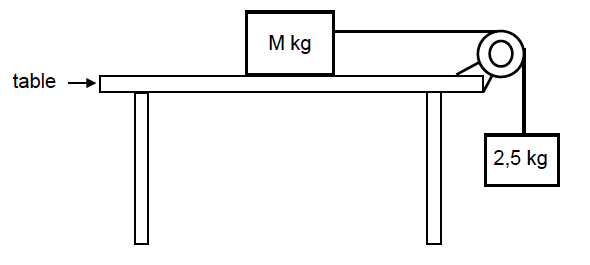GR 12 Activity

1.1. Two blocks of mass M kg and 2,5 kg respectively are connected by a light, inextensible string. The string runs over a light, frictionless pulley, as shown in the diagram below.
The blocks are stationary.1. State Newton’s THIRD law in words. (2)
2. Calculate the tension in the string. (3)

The coefficient of static friction (μs) between the unknown mass M and the surface of the table is 0,2.

3. Calculate the minimum value of M that will prevent the blocks from moving. (5)

The block of unknown mass M is now replaced with a block of mass 5 kg. The 2,5 kg block now accelerates downwards. The coefficient of kinetic friction (µk) between the 5 kg block and the surface of the table is 0,15.

4. Calculate the magnitude of the acceleration of the 5 kg block. (5)

1.2. A small hypothetical planet X has a mass of 6,5 x 1020 kg and a radius of 550 km.
Calculate the gravitational force (weight) that planet X exerts on a 90 kg rock on this planet’s surface. (4)


Q2

A bullet of mass 20 g is fired from a stationary rifle of mass 3 kg. Assume that the bullet moves horizontally. Immediately after firing, the rifle recoils (moves back) with a velocity of 1,4 m∙s-1.

1. Calculate the speed at which the bullet leaves the rifle. (4)

The bullet strikes a stationary 5 kg wooden block fixed to a flat, horizontal table. The bullet is brought to rest after travelling a distance of 0,4 m into the block. Refer to the diagram below.2. Calculate the magnitude of the average force exerted by the block on the bullet. (5)

3. How does the magnitude of the force calculated in QUESTION 2 compare to the magnitude of the force exerted by the bullet on the block? Write down only LARGER THAN, SMALLER THAN or THE SAME. (1)                                                                                               

Q3

A 5 kg block, resting on a rough horizontal table, is connected by a light inextensible string passing over a light frictionless pulley to another block of mass 2 kg. The 2 kg block hangs vertically as shown in the diagram below.A force of 60 N is applied to the 5 kg block at an angle of 10o to the horizontal, causing the block to accelerate to the left.
The coefficient of kinetic friction between the 5 kg block and the surface of the table is 0,5. Ignore the effects of air friction.

1. Draw a labelled free-body diagram showing ALL the forces acting on the 5 kg block. (5)

2. Calculate the magnitude of the:

2.1. Vertical component of the 60 N force (2)
2.2.Horizontal component of the 60 N force (2)
2.3. State Newton’s Second Law of Motion in words. (2)

Calculate the magnitude of the:

1.Normal force acting on the 5 kg block (2)
2.Tension in the string connecting the two blocks (7)


The letters A to F in the table below represent six organic compounds.1.1. Write down the:
1. NAME of the functional group of compound B NAME of the functional group of compound B (1)
2.Homologous series to which compound C belongs (1)
3.Type of polymerisation reaction that produces compound F (1)
1.2. Write down the IUPAC name of:
1. The monomer used to prepare compound F (1)
2. Compound C (2)
3. Compound D (2)
1.3. Write down the NAME or FORMULA of each product formed during the complete combustion of compound D. (2)
1.4. Write down the structural formula of:
1.Compound B (2)
2.A CHAIN ISOMER of compound A (2)

A laboratory assistant uses bromine water to distinguish between compounds D and E. She adds bromine water to a sample of each in two different test tubes. She observes that the one compound decolourises the bromine water immediately, whilst the other one only reacts after placing the test tube in direct sunlight.

1.5. Write down the:
1. Letter (D or E) of the compound that will immediately decolourise the bromine water (1)
2. Name of the type of reaction that takes place in the test tube containing compound D (1)
3. Structural formula of the organic product formed in the test tube containing compound E (2)                                                                                                                                                                

5 Comments on “GR 12 Activity”

1.Joseph Hlongwane

Thanks for the exercise, l gave it to my students.

2.Elizabeth Amukwaya

Please do some revision questions on the topic of Electricity

3.Phapama

This grade12 activities helped me alot ”especially tht ‘test yourslf” coz i’ve always been practisng here and also shown my friends and they lke it

4.Solomon Tyobeka

That’s wonderful!

This site uses Akismet to reduce spam. Learn how your comment data is processed.